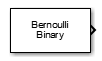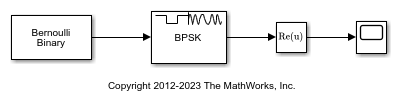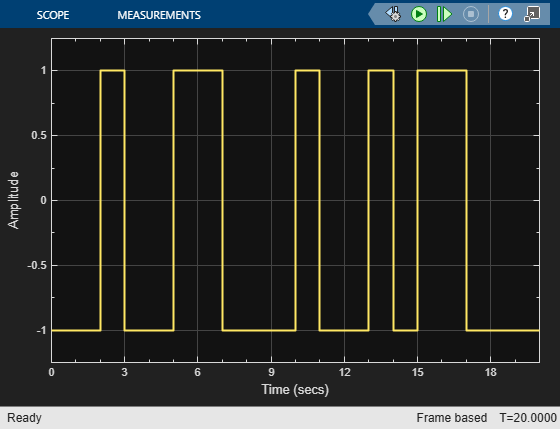Documentation

# Bernoulli Binary Generator

Generate Bernoulli-distributed random binary numbers

## Library

Random Data Sources sublibrary of Comm Sources## Description

The Bernoulli Binary Generator block generates random binary numbers using a Bernoulli distribution. The Bernoulli distribution with parameter p produces zero with probability p and one with probability 1-p. The Bernoulli distribution has mean value 1-p and variance p(1-p). The Probability of a zero parameter specifies p, and can be any real number between zero and one.

### Attributes of Output Signal

The output signal can be a column or row vector, a two-dimensional matrix, or a scalar. The number of rows in the output signal corresponds to the number of samples in one frame and is determined by the Samples per frame parameter. The number of columns in the output signal corresponds to the number of channels and is determined by the number of elements in the Probability of a zero parameter. See Sources and Sinks in Communications Toolbox™ User's Guide for more details.

## Parameters

Probability of a zero

The probability with which a zero output occurs. Specify the probability as a scalar or row vector whose elements are real numbers between 0 and 1. The number of elements in the Probability of a zero parameter correspond to the number of independent channels output from the block.

Source of initial seed

The source of the initial seed for the random number generator. Specify the source as either `Auto` or `Parameter`. When set to `Auto`, the block uses the global random number stream.

### Note

When Source of initial seed is `Auto` in `Code generation` mode, the random number generator uses an initial seed of zero. Therefore, the block generates the same random numbers each time it is started. Use `Interpreted execution` to ensure that the model uses different initial seeds. If ```Interpreted execution``` is run in `Rapid accelerator` mode, then it behaves the same as ```Code generation``` mode.

Initial seed

The initial seed value for the random number generator. Specify the seed as a nonnegative integer scalar. Initial seed is available when the Source of initial seed parameter is set to `Parameter`.

Sample time

The time between each sample of a column of the output signal.

Samples per frame

The number of samples per frame in one channel of the output signal. Specify Samples per frame as a positive integer scalar.

### Note

The time between output updates is equal to the product of Samples per frame and Sample time. For example, if Sample time and Samples per frame equal one, the block outputs a binary sample every second. If Samples per frame is increased to 10, then a 10-by-1 vector is output every 10 seconds. This ensures that the equivalent output rate is not dependent on the Samples per frame parameter.

Output data type

The output type of the block can be specified as a `boolean`, `uint8`, `uint16`, `uint32`, `single`, or `double`. The default is `double`.

Simulate using

Select the simulation mode.

```Code generation```

On the first model run, simulate and generate code. If the structure of the block does not change, subsequent model runs do not regenerate the code.

If the simulation mode is ```Code generation```, System objects corresponding to the blocks accept a maximum of nine inputs.

```Interpreted execution```

Simulate model without generating code. This option results in faster start times but can slow subsequent simulation performance.

## Examples

expand all

Open the Bernoulli generator model. The model generates binary data, applies BPSK modulation, and displays the output.Run the model.